阈值化处理 - CSDN

• ## OpenCV阈值化处理

千次阅读 2018-06-21 14:49:14
图像的阈值化就是利用图像像素点分布规律，设定阈值进行像素点分割，进而得到图像的二值图像。图像阈值化操作有多种方法，常用方法有经典的OTSU、固定阈值、自适应阈值、双阈值及半阈值化操作。这里对各种阈值化操作进行一个总结。

OTSU阈值化

我们知道一幅灰度图像，可以计算它的颜色平均值，或者更进一步，可以计算出灰度直方图。我们可以把这幅图的灰度平均值为M，任意选取一个灰度值t，则可以将这个直方图分成前后两部分。我们称这两部分分别为A和B，对应的就是前景色和背景色。这两部分各自的平均值为M0${M}_{0}$$M_0$和M1${M}_{1}$$M_1$。A部分里的像素站总像素数的比例为p0${p}_{0}$$p_0$，B部分里的像素数站总像素数的比例为p1${p}_{1}$$p_1$。Nobuyuki Ostu给出的类间方差定义为：
ICV=p0∗(M0−M)2+p1∗(M1−M)2)(1)$\begin{array}{}\text{(1)}& ICV={p}_{0}\ast \left({M}_{0}-M{\right)}^{2}+{p}_{1}\ast \left({M}_{1}-M{\right)}^{2}\right)\end{array}$
ICV = p_0*(M_0 - M)^2 + p_1*(M_1 - M)^2\tag 1)

展开后如下：
p0M20−2p0M0M+p0M2+p1M21−2p1M1M+P1M2${p}_{0}{M}_{0}^{2}-2{p}_{0}{M}_{0}M+{p}_{0}{M}^{2}+{p}_{1}{M}_{1}^{2}-2{p}_{1}{M}_{1}M+{P}_{1}{M}^{2}$
p_0 M_0^2 - 2p_0M_0M+p_0M^2+ p_1M_1^2-2p_1M_1M+P_1M^2

又：
p0+p1=1,p0M0+p1M1=M${p}_{0}+{p}_{1}=1,{p}_{0}{M}_{0}+{p}_{1}{M}_{1}=M$ p_0 + p_1 = 1,p_0M_0 + p_1M_1 = M
带入(1)得如下：
p0p1(M0−M1)2${p}_{0}{p}_{1}\left({M}_{0}-{M}_{1}{\right)}^{2}$
p_0p_1(M_0-M_1)^2

其实现代码如下：

#include "opencv2/core/core.hpp"
#include "opencv2/imgproc/imgproc.hpp"
#include "opencv2/highgui/highgui.hpp"
#include <iostream>
#include <string>
#include <cmath>

using namespace cv;
int Otsu(Mat matSrc)
{
if (CV_8UC1 != matSrc.type())
return -1;
int nCols = matSrc.cols;
int nRows = matSrc.rows;
int nPixelNum = nCols * nRows;
// 初始化
int pixelNum;
double probability;
for (int i = 0; i < 256; i++)
{
pixelNum[i] = 0;
probability[i] = 0.0;
}
// 统计像素数和频率
for (int j = 0; j < nRows; j++)
{
for (int i = 0; i < nCols; i++)
{
pixelNum[matSrc.at<uchar>(j, i)]++;
}
}
for (int i = 0; i < 256; i++)
{
probability[i] = (double)0.1*pixelNum[i] / nPixelNum;
}
// 计算
int nThreshold = 0;          // 最佳阈值
double dMaxDelta = 0.0;      // 最大类间方差
double dMean_0 = 0.0;        // 左边平均值
double dMean_1 = 0.0;        // 右边平均值
double dDelta = 0.0;         // 类间方差
double dMean_0_temp = 0.0;   // 左边平均值中间值
double dMean_1_temp = 0.0;   // 右边平均值中间值
double dProbability_0 = 0.0;       // 左边频率值
double dProbability_1 = 0.0;       // 右边频率值
for (int j = 0; j < 256; j++)
{
for (int i = 0; i < 256; i++)
{
if (i < j)// 前半部分
{
dProbability_0 += probability[i];
dMean_0_temp += i * probability[i];
}
else      // 后半部分
{
dProbability_1 += probability[i];
dMean_1_temp += i * probability[i];
}
}
// 计算平均值
// fMean_0_teamp计算的是前半部分的灰度值的总和除以总像素数，
// 所以要除以前半部分的频率才是前半部分的平均值，后半部分同样
dMean_0 = dMean_0_temp / dProbability_0;
dMean_1 = dMean_1_temp / dProbability_1;
dDelta = (double)(dProbability_0 * dProbability_1 * pow((dMean_0 - dMean_1), 2));
if (dDelta > dMaxDelta)
{
dMaxDelta = dDelta;
nThreshold = j;
}
// 相关参数归零
dProbability_0 = 0.0;
dProbability_1 = 0.0;
dMean_0_temp = 0.0;
dMean_1_temp = 0.0;
dMean_0 = 0.0;
dMean_1 = 0.0;
dDelta = 0.0;
}
return nThreshold;
}

测试代码如下：

int main()
{
std::string strPath = "D:\\MyDocuments\\My Pictures\\OpenCV\\";
Mat matSrc = imread(strPath + "shrimp.jpg");
if (matSrc.empty())
return -1;
int nCols = matSrc.cols;
int nRows = matSrc.rows;
Mat matGray;
cvtColor(matSrc, matGray, CV_BGR2GRAY);
imshow("gray", matGray);
int nOstuThreshold = Otsu(matGray);
std::cout << nOstuThreshold << std::endl;
Mat matOstu = Mat::zeros(matGray.rows, matGray.cols, CV_8UC1);
// 用得到的值进行二值化处理
for (int j = 0; j < nRows; j++)
{
for (int i = 0; i < nCols; i++)
{
if (matGray.at<uchar>(j, i) < nOstuThreshold)
{
// matOstu.at<uchar>(j, i) = matGray.at<uchar>(j, i);
matOstu.at<uchar>(j, i) = matGray.at<uchar>(j, i);
}
else
{
// matOstu.at<uchar>(j, i) = matGray.at<uchar>(j, i);
matOstu.at<uchar>(j, i) = 0;
}
}
}
imshow("ostu", matOstu);
imwrite(strPath + "ostu.jpg", matOstu);
waitKey();
return 0;
}

原图：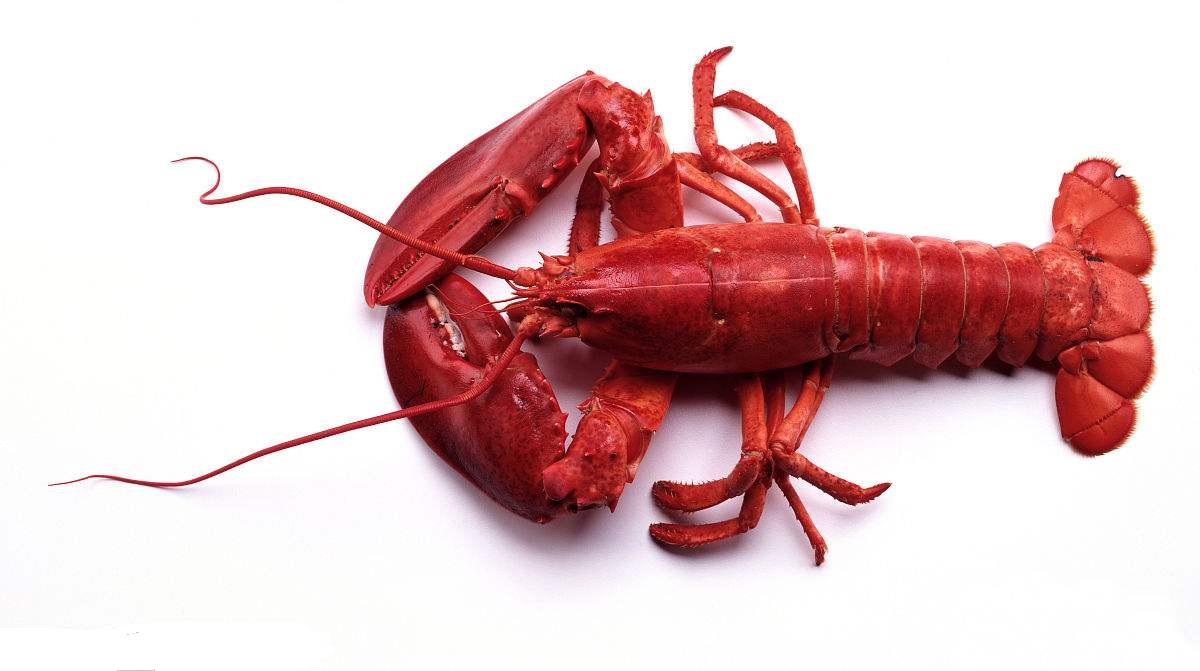阈值处理后：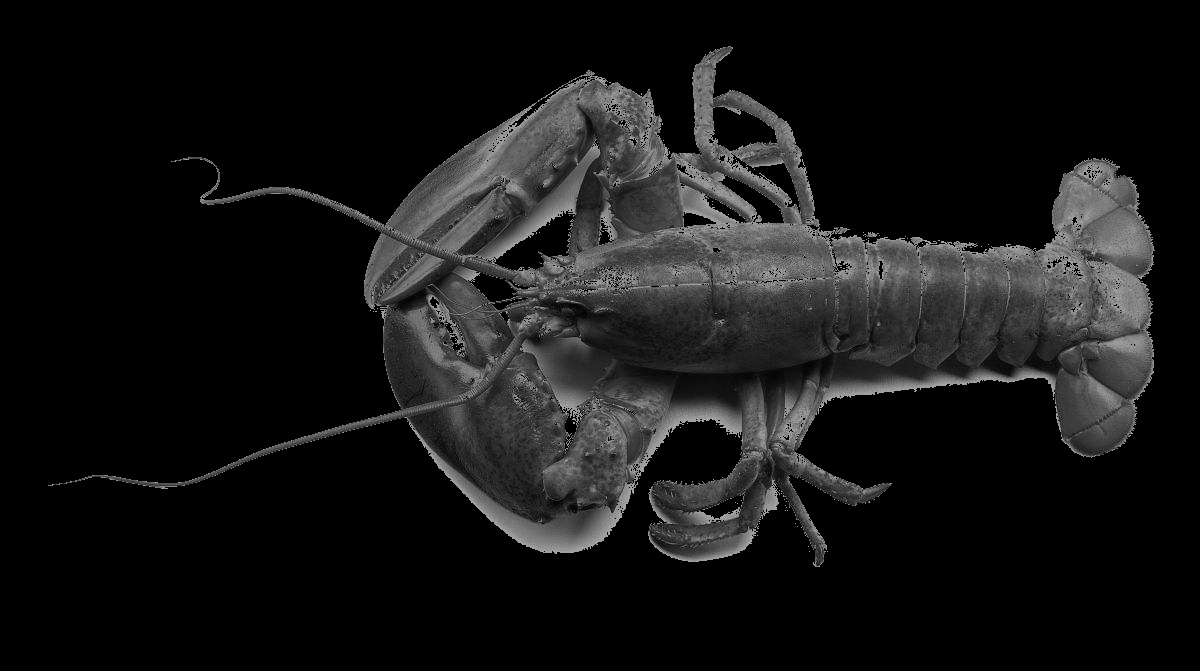固定阈值化

OpenCV中提供了阈值化函数threshold，该函数有5中阈值化类型参数。简单说就是用一个固定阈值来分割图像。至于分割方式有多种。分别对应不同的阈值化方式。其函数原型如下：

double threshold(InputArray src, OutputArray dst, double thresh, double maxval, int type)

参数src表示原图像数组（单通道，8位或32位浮点数据）;dst表示输出图像组；thresh表示阈值大小;maxval表示预设最大值（THRESH_BINARY或THRESH_BINARY_INV时有用）;type表示阈值化处理的类型设置。type的各种类型如下：

THRESH_BINARY二进制阈值化

将灰度值大于thresh的设置为maxval，不大于thresh设置为0。灰度计算式如下：
dst(x,y)={maxval0src(x,y)>threshotherwise$dst\left(x,y\right)=\left\{\begin{array}{ll}maxval& src\left(x,y\right)>thresh\\ 0& otherwise\end{array}$dst(x, y) = \begin{cases}
maxval&src(x, y)>thresh\\
0&otherwise
\end{cases}

THRESH_BINARY_INV反二进制阈值化

将灰度值大于thresh的设置为0，不大于thresh设置为maxval。灰度计算式如下：
dst(x,y)={maxval0src(x,y)≤threshotherwise$dst\left(x,y\right)=\left\{\begin{array}{ll}maxval& src\left(x,y\right)\le thresh\\ 0& otherwise\end{array}$dst(x, y) = \begin{cases}
maxval&src(x, y)\leq thresh\\
0&otherwise
\end{cases}

THRESH_TRUNC截断阈值化

将灰度值大于thresh的设置为threshold，不大于thresh的灰度值不变。灰度计算式如下：
dst(x,y)={thresholdsrc(x,y)src(x,y)>threshotherwise$dst\left(x,y\right)=\left\{\begin{array}{ll}threshold& src\left(x,y\right)>thresh\\ src\left(x,y\right)& otherwise\end{array}$dst(x, y) = \begin{cases}
threshold &src(x, y)> thresh\\
src(x, y)&otherwise
\end{cases}

THRESH_TOZERO阈值化为0

将灰度值大于thresh的不变，不大于thresh的灰度值设置为0。灰度计算式如下：
dst(x,y)={src(x,y)0src(x,y)>threshotherwise$dst\left(x,y\right)=\left\{\begin{array}{ll}src\left(x,y\right)& src\left(x,y\right)>thresh\\ 0& otherwise\end{array}$dst(x, y) = \begin{cases}
src(x, y) &src(x, y)> thresh\\
0&otherwise
\end{cases}

THRESH_TOZERO_INV反阈值化为0

将灰度值大于thresh的不变，不大于thresh的灰度值设置为0。灰度计算式如下：
dst(x,y)={src(x,y)0src(x,y)≤threshotherwise$dst\left(x,y\right)=\left\{\begin{array}{ll}src\left(x,y\right)& src\left(x,y\right)\le thresh\\ 0& otherwise\end{array}$dst(x, y) = \begin{cases}
src(x, y) &src(x, y)\leq thresh\\
0&otherwise
\end{cases}
试例代码如下：

#include "opencv2/core/core.hpp"
#include "opencv2/imgproc/imgproc.hpp"
#include "opencv2/highgui/highgui.hpp"
#include <iostream>
#include <string>
#include <cmath>

using namespace cv;
int main()
{
std::string strPath = "D:\\MyDocuments\\My Pictures\\OpenCV\\";
Mat matSrc = imread(strPath + "shrimp.jpg");
Mat matGray, matThresh;
cvtColor(matSrc, matGray, CV_BGR2GRAY);
threshold(matGray, matThresh, 156, 255, CV_THRESH_TOZERO_INV);
imshow("gray", matGray);
imshow("thresh", matThresh);
imwrite(strPath + "thresh.jpg", matThresh);
waitKey();
return 0;
}

原图：阈值处理后的图：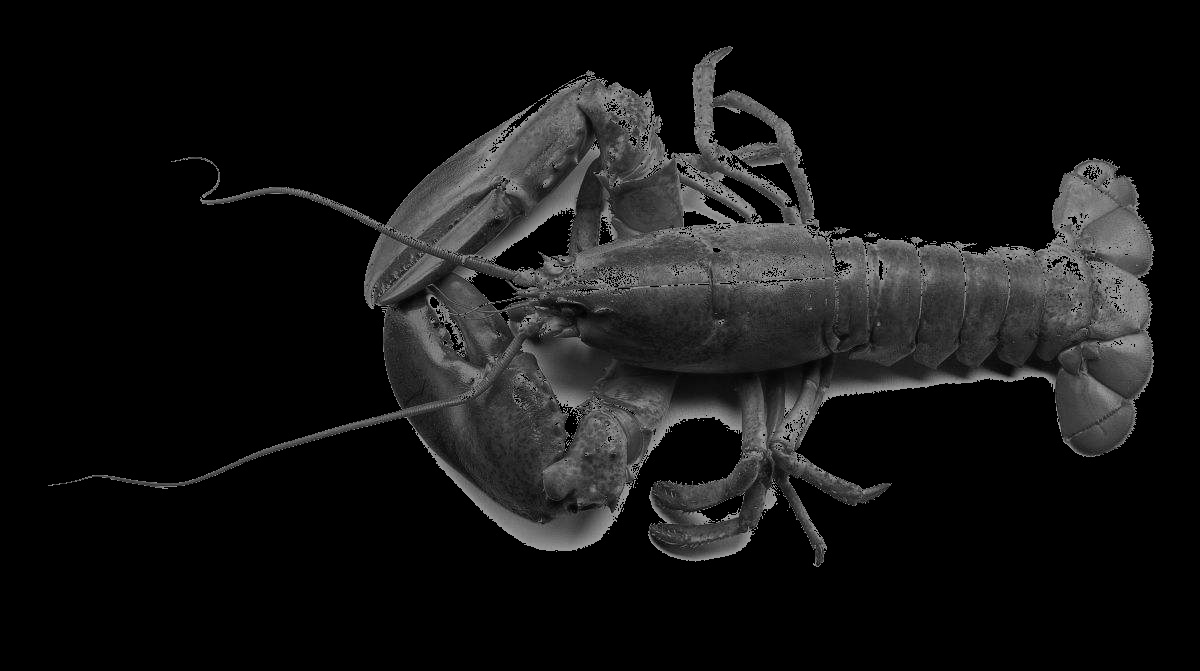自适应阈值化

在图像阈值化操作中，我们更关心的是从二值化图像中分离目标区域和背景区域，仅仅通过固定阈值很难达到理想的分割效果。在图片中的灰度是不均匀的，所以通常情况下图片中不同区域的阈值时不一样的。在不同局部选取阈值的方法有多种。在OpenCV中实现了两种方法：1）局部邻域块的均值；2）局部邻域块的高斯加权和。其函数原型如下：

void adaptiveThreshold(InoutArray src, OutputArray dst, double maxValue, int adaptiveMethod, int thresholdType, int blockSize, double C)

src表示源图像数组；

dst表示输出图像组；

maxValue表示预设满足条件最大值；

thresholdType表示阈值类型THRESH_BINARY或THRESH_BINARY_INV;

blockSize表示邻域块大小，用来计算区域阈值，一般选择3、5、7……;

参数C表示常数，它是一个从均匀或加权均值提取的常数，可以是负数。
试例代码如下：

#include "opencv2/core/core.hpp"
#include "opencv2/imgproc/imgproc.hpp"
#include "opencv2/highgui/highgui.hpp"
#include <iostream>
#include <string>
#include <cmath>

using namespace cv;
{
std::string strPath = "D:\\MyDocuments\\My Pictures\\OpenCV\\";
Mat matSrc = imread(strPath + "panda.jpg");
cvtColor(matSrc, matGray, CV_BGR2GRAY);

imshow("gray", matGray);

waitKey();
return 0;
}

原图：阈值处理后的图：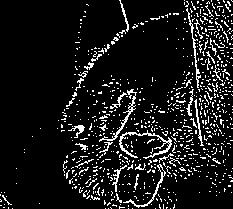展开全文• 原理摘自：...OpenCV中的阈值(threshold)函数： threshold 的运用。 注意：本节的解释出自Bradski与Kaehler的书籍 Learning O

原理摘自：http://www.opencv.org.cn/opencvdoc/2.3.2/html/doc/tutorials/imgproc/threshold/threshold.html

本节简介：
OpenCV中的阈值(threshold)函数：  threshold  的运用。

最简单的图像分割的方法。

应用举例：从一副图像中利用阈值分割出我们需要的物体部分（当然这里的物体可以是一部分或者整体）。这样的图像分割方法是基于图像中物体与背景之间的灰度差异，而且此分割属于像素级的分割。

为了从一副图像中提取出我们需要的部分，应该用图像中的每一个像素点的灰度值与选取的阈值进行比较，并作出相应的判断。（注意：阈值的选取依赖于具体的问题。即：物体在不同的图像中有可能会有不同的灰度值。

一旦找到了需要分割的物体的像素点，我们可以对这些像素点设定一些特定的值来表示。（例如：可以将该物体的像素点的灰度值设定为：‘0’（黑色）,其他的像素点的灰度值为：‘255’（白色）；当然像素点的灰度值可以任意，但最好设定的两种颜色对比度较强，方便观察结果）。OpenCV中提供了阈值（threshold）函数： threshold  。

这个函数有5种阈值化类型，在接下来的章节中将会具体介绍。

为了解释阈值分割的过程，我们来看一个简单有关像素灰度的图片，该图如下。该图中的蓝色水平线代表着具体的一个阈值。阈值类型1：二进制阈值化

该阈值化类型如下式所示:解释：在运用该阈值类型的时候，先要选定一个特定的阈值量，比如：125，这样，新的阈值产生规则可以解释为大于125的像素点的灰度值设定为最大值(如8位灰度值最大为255)，灰度值小于125的像素点的灰度值设定为0。阈值类型2：反二进制阈值化

该阈值类型如下式所示：解释：该阈值化与二进制阈值化相似，先选定一个特定的灰度值作为阈值，不过最后的设定值相反。（在8位灰度图中，例如大于阈值的设定为0，而小于该阈值的设定为255）。阈值类型3：截断阈值化

该阈值化类型如下式所示：解释：同样首先需要选定一个阈值，图像中大于该阈值的像素点被设定为该阈值，小于该阈值的保持不变。（例如：阈值选取为125，那小于125的阈值不改变，大于125的灰度值（230）的像素点就设定为该阈值）。阈值类型4：阈值化为0

该阈值类型如下式所示：解释：先选定一个阈值，然后对图像做如下处理：1 像素点的灰度值大于该阈值的不进行任何改变；2 像素点的灰度值小于该阈值的，其灰度值全部变为0。阈值类型5：反阈值化为0

该阈值类型如下式所示：解释：原理类似于0阈值，但是在对图像做处理的时候相反，即：像素点的灰度值小于该阈值的不进行任何改变，而大于该阈值的部分，其灰度值全部变为0。部分代码：
// ConsoleApplication3_6_23.cpp : Defines the entry point for the console application.
//

#include "stdafx.h"
#include<opencv2/opencv.hpp>
#include<iostream>
using namespace std;
using namespace cv;

Mat src,gray,dst;
int thr_value = 0;
int thr_type = 0;

const int max_type = 4;
const int max_value = 255;
const int max_binary_value = 255;

char *windowName = "Demo";
void Image_thred(int,void*);
int _tmain(int argc, _TCHAR* argv[])
{

if(!src.data)
return -1;

cvtColor(src,gray,CV_RGB2GRAY);
namedWindow("灰度图",CV_WINDOW_AUTOSIZE);
imshow("灰度图",gray);

namedWindow(windowName,CV_WINDOW_AUTOSIZE);
createTrackbar("Type: \n 0: Binary \n 1: Binary Inverted \n 2: Truncate \n 3: To Zero \n 4: To Zero Inverted",
windowName,&thr_type,max_type,Image_thred);
createTrackbar("Value",
windowName,&thr_value,max_value,Image_thred);

Image_thred(0,0);
waitKey(0);
return 0;
}

void Image_thred(int,void*)
{
/* 0: 二进制阈值
1: 反二进制阈值
2: 截断阈值
3: 0阈值
4: 反0阈值
*/
threshold(gray,dst,thr_value,max_binary_value,thr_type);
imshow(windowName,dst);
}
关键函数解释：

threshold( src_gray, dst, threshold_value, max_BINARY_value,threshold_type );
src_gray : 输入的灰度图像的地址。dst : 输出图像的地址。threshold_value : 进行阈值操作时阈值的大小。max_BINARY_value : 设定的最大灰度值（该参数运用在二进制与反二进制阈值操作中）。threshold_type : 阈值的类型。从上面提到的5种中选择出的结果。

展开全文opencv
• 该系列文章是讲解Python OpenCV图像处理...本篇文章主要讲解Python调用OpenCV实现图像阈值化处理操作，包括二进制阈值化、反二进制阈值化、截断阈值化、反阈值化为0、阈值化为0。全文均是基础知识，希望对您有所帮助。
该系列文章是讲解Python OpenCV图像处理知识，前期主要讲解图像入门、OpenCV基础用法，中期讲解图像处理的各种算法，包括图像锐化算子、图像增强技术、图像分割等，后期结合深度学习研究图像识别、图像分类应用。希望文章对您有所帮助，如果有不足之处，还请海涵~
该系列在github所有源代码：https://github.com/eastmountyxz/ImageProcessing-Python
PS：请求帮忙点个Star，哈哈，第一次使用Github，以后会分享更多代码，一起加油。
同时推荐作者的C++图像系列知识：
[数字图像处理] 一.MFC详解显示BMP格式图片
[数字图像处理] 二.MFC单文档分割窗口显示图片
[数字图像处理] 三.MFC实现图像灰度、采样和量化功能详解
[数字图像处理] 四.MFC对话框绘制灰度直方图
[数字图像处理] 五.MFC图像点运算之灰度线性变化、灰度非线性变化、阈值化和均衡化处理详解
[数字图像处理] 六.MFC空间几何变换之图像平移、镜像、旋转、缩放详解
[数字图像处理] 七.MFC图像增强之图像普通平滑、高斯平滑、Laplacian、Sobel、Prewitt锐化详解
前文参考：
[Python图像处理] 一.图像处理基础知识及OpenCV入门函数
[Python图像处理] 二.OpenCV+Numpy库读取与修改像素
[Python图像处理] 三.获取图像属性、兴趣ROI区域及通道处理
[Python图像处理] 四.图像平滑之均值滤波、方框滤波、高斯滤波及中值滤波
[Python图像处理] 五.图像融合、加法运算及图像类型转换
[Python图像处理] 六.图像缩放、图像旋转、图像翻转与图像平移
本篇文章主要讲解Python调用OpenCV实现图像阈值化处理操作，包括二进制阈值化、反二进制阈值化、截断阈值化、反阈值化为0、阈值化为0。全文均是基础知识，希望对您有所帮助。
1.阈值化
2.二进制阈值化
3.反二进制阈值化
4.截断阈值化
5.反阈值化为0
6.阈值化为0
PS：文章参考自己以前系列图像处理文章及OpenCV库函数，同时部分参考网易云视频，推荐大家去学习。同时，本篇文章涉及到《计算机图形学》基础知识，请大家下来补充。
PSS：2019年1~2月作者参加了CSDN2018年博客评选，希望您能投出宝贵的一票。我是59号，Eastmount，杨秀璋。投票地址：https://bss.csdn.net/m/topic/blog_star2018/index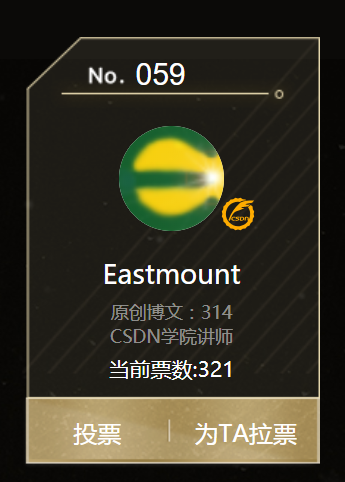五年来写了314篇博客，12个专栏，是真的热爱分享，热爱CSDN这个平台，也想帮助更多的人，专栏包括Python、数据挖掘、网络爬虫、图像处理、C#、Android等。现在也当了两年老师，更是觉得有义务教好每一个学生，让贵州学子好好写点代码，学点技术，"师者，传到授业解惑也"，提前祝大家新年快乐。2019我们携手共进，为爱而生。

一. 阈值化
（注：该部分参考作者的论文《基于苗族服饰的图像锐化和边缘提取技术研究》）
图像的二值化或阈值化（Binarization）旨在提取图像中的目标物体，将背景以及噪声区分开来。通常会设定一个阈值T，通过T将图像的像素划分为两类：大于T的像素群和小于T的像素群。
灰度转换处理后的图像中，每个像素都只有一个灰度值，其大小表示明暗程度。二值化处理可以将图像中的像素划分为两类颜色，常用的二值化算法如公式1所示：
$\begin{cases} Y=0，gray=T\\ \end{cases}$
当灰度Gray小于阈值T时，其像素设置为0，表示黑色；当灰度Gray大于或等于阈值T时，其Y值为255，表示白色。
Python OpenCV中提供了阈值函数threshold()实现二值化处理，其公式及参数如下图所示：
retval, dst = cv2.threshold(src, thresh, maxval, type)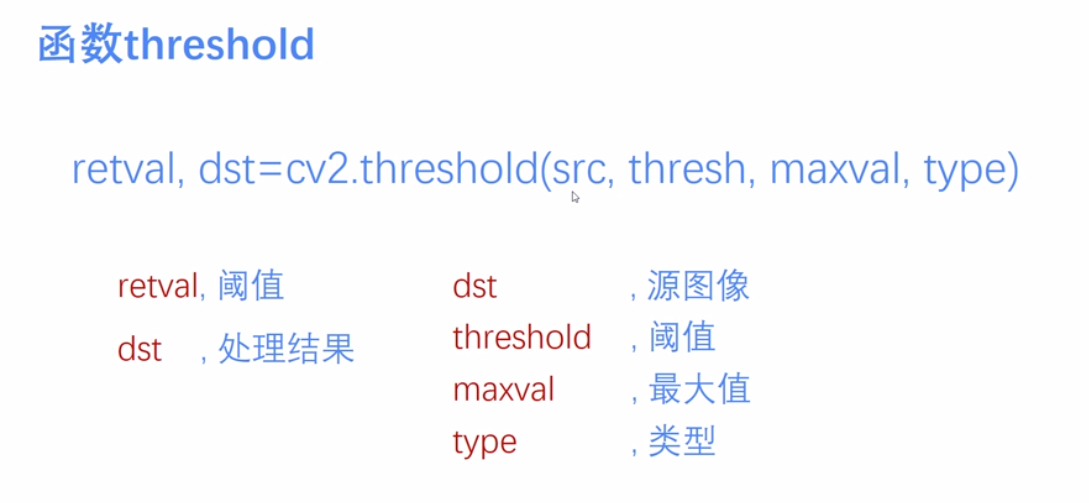常用的方法如下表所示，其中函数中的参数Gray表示灰度图，参数127表示对像素值进行分类的阈值，参数255表示像素值高于阈值时应该被赋予的新像素值，最后一个参数对应不同的阈值处理方法。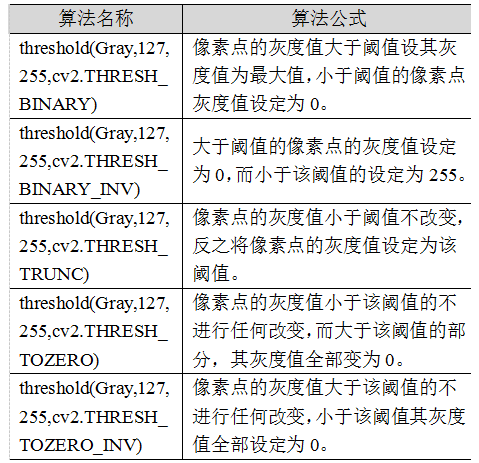对应OpenCV提供的五张图如下所示，第一张为原图，后面依次为：二进制阈值化、反二进制阈值化、截断阈值化、反阈值化为0、阈值化为0。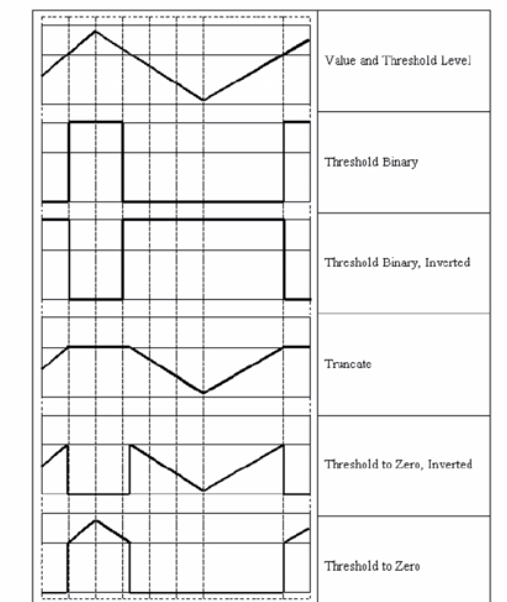二值化处理广泛应用于各行各业，比如生物学中的细胞图分割、交通领域的车牌设别等。在文化应用领域中，通过二值化处理将所需民族文物图像转换为黑白两色图，从而为后面的图像识别提供更好的支撑作用。下图表示图像经过各种二值化处理算法后的结果，其中“BINARY”是最常见的黑白两色处理。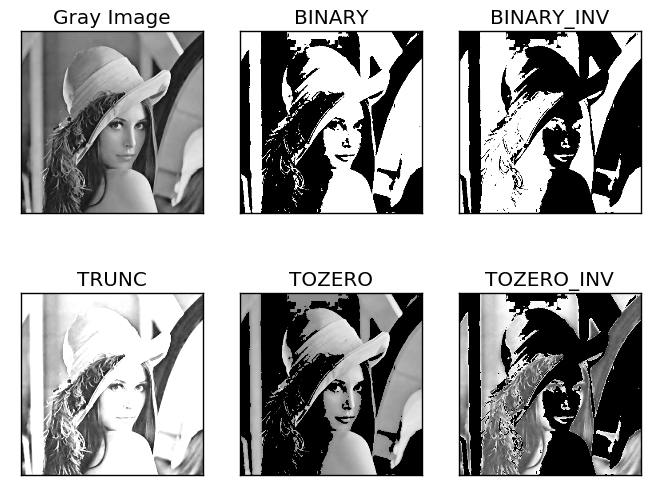二. 二进制阈值化
该方法先要选定一个特定的阈值量，比如127。新的阈值产生规则如下：
$dst(x,y) = \begin{cases} maxVal， if src(x,y)>thresh\\ 0，otherwise\\ \end{cases}$
(1) 大于等于127的像素点的灰度值设定为最大值（如8位灰度值最大为255）
(2) 灰度值小于127的像素点的灰度值设定为0
例如，163->255，86->0，102->0，201->255。
关键字为 cv2.THRESH_BINARY，完整代码如下：
#encoding:utf-8
import cv2
import numpy as np

#读取图片

#灰度图像处理
GrayImage = cv2.cvtColor(src,cv2.COLOR_BGR2GRAY)

#二进制阈值化处理
r, b = cv2.threshold(GrayImage, 127, 255, cv2.THRESH_BINARY)
print r

#显示图像
cv2.imshow("src", src)
cv2.imshow("result", b)

#等待显示
cv2.waitKey(0)
cv2.destroyAllWindows()

输出为两个返回值，r为127，b为处理结果（大于127设置为255，小于设置为0）。如下图所示：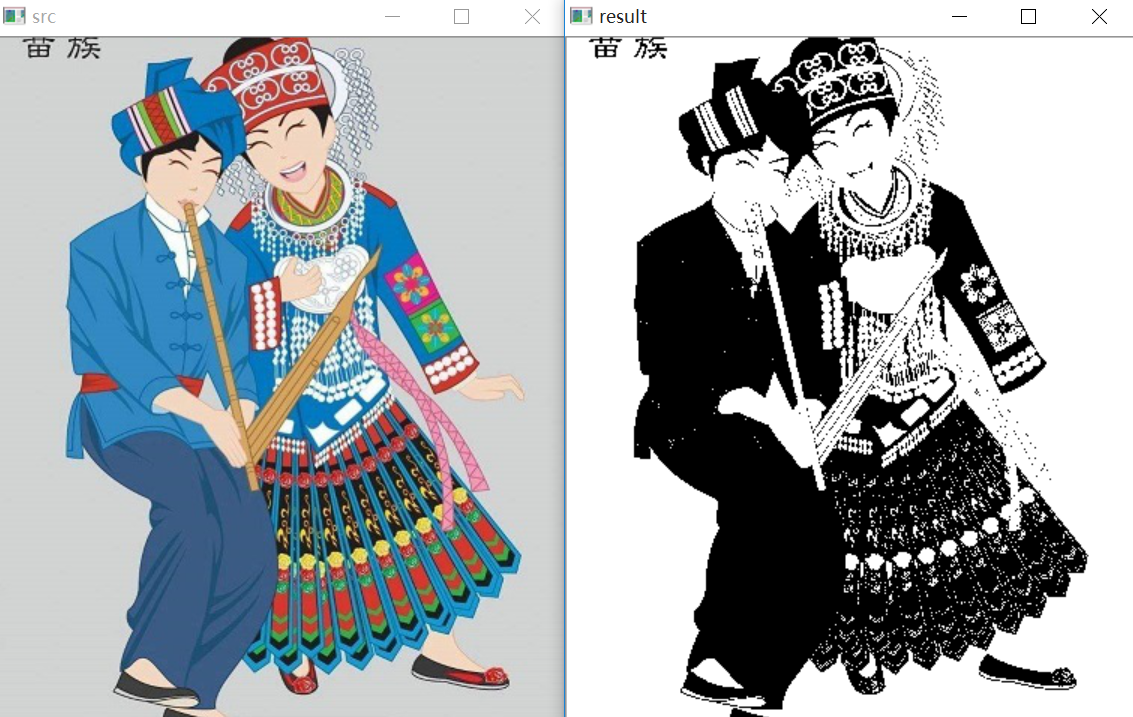三. 反二进制阈值化
该方法与二进制阈值化方法相似，先要选定一个特定的灰度值作为阈值，比如127。新的阈值产生规则如下：
$dst(x,y) = \begin{cases} 0， if src(x,y)>thresh\\ maxVal，otherwise\\ \end{cases}$
(1) 大于127的像素点的灰度值设定为0（以8位灰度图为例）
(2) 小于该阈值的灰度值设定为255
例如，163->0，86->255，102->255，201->0。
关键字为 cv2.THRESH_BINARY_INV，完整代码如下：
#encoding:utf-8
import cv2
import numpy as np

#读取图片

#灰度图像处理
GrayImage = cv2.cvtColor(src,cv2.COLOR_BGR2GRAY)

#反二进制阈值化处理
r, b = cv2.threshold(GrayImage, 127, 255, cv2.THRESH_BINARY_INV)
print r

#显示图像
cv2.imshow("src", src)
cv2.imshow("result", b)

#等待显示
cv2.waitKey(0)
cv2.destroyAllWindows()

输出结果如下图所示：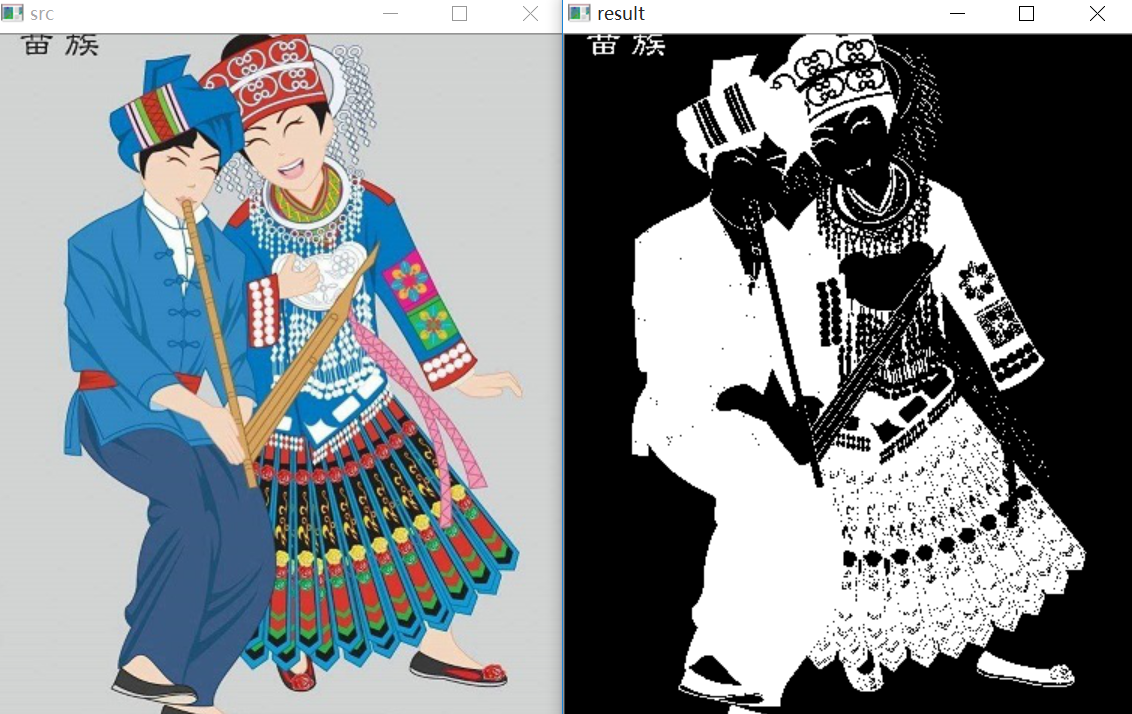该方法得到的结果正好与二进制阈值化方法相反，亮色元素反而处理为黑色，暗色处理为白色。

四. 截断阈值化
该方法需要选定一个阈值，图像中大于该阈值的像素点被设定为该阈值，小于该阈值的保持不变，比如127。新的阈值产生规则如下：
$dst(x,y) = \begin{cases} threshold， if src(x,y)>thresh\\ src(x,y)，otherwise\\ \end{cases}$
(1) 大于等于127的像素点的灰度值设定为该阈值127
(2) 小于该阈值的灰度值不改变
例如，163->127，86->86，102->102，201->127。
关键字为 cv2.THRESH_TRUNC，完整代码如下：
#encoding:utf-8
import cv2
import numpy as np

#读取图片

#灰度图像处理
GrayImage = cv2.cvtColor(src,cv2.COLOR_BGR2GRAY)

#截断阈值化处理
r, b = cv2.threshold(GrayImage, 127, 255, cv2.THRESH_TRUNC)
print r

#显示图像
cv2.imshow("src", src)
cv2.imshow("result", b)

#等待显示
cv2.waitKey(0)
cv2.destroyAllWindows()

输出结果如下图所示：：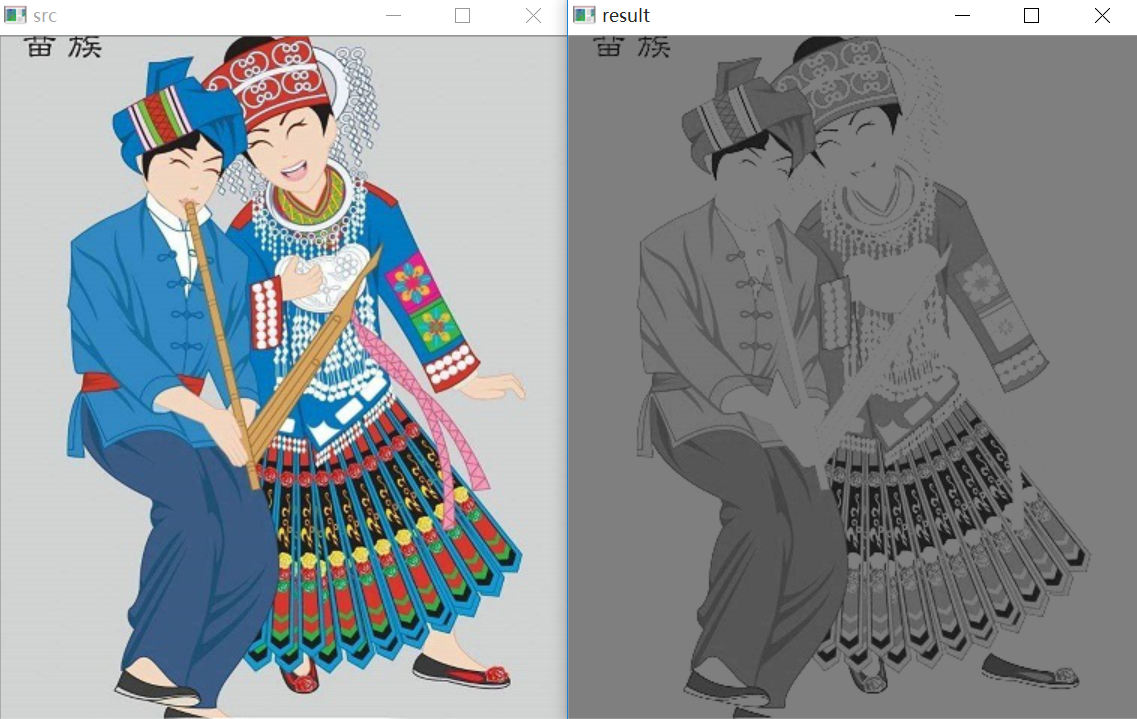该处理方法相当于把图像中比较亮（大于127，偏向于白色）的像素值处理为阈值。

五. 反阈值化为0
该方法先选定一个阈值，比如127，接着对图像的灰度值进行如下处理：
$dst(x,y) = \begin{cases} 0， if src(x,y)>thresh\\ src(x,y)，otherwise\\ \end{cases}$
(1) 大于等于阈值127的像素点变为0
(2) 小于该阈值的像素点值保持不变
例如，163->0，86->86，102->102，201->0。
关键字为 cv2.THRESH_TOZERO_INV，完整代码如下：
#encoding:utf-8
import cv2
import numpy as np

#读取图片

#灰度图像处理
GrayImage = cv2.cvtColor(src,cv2.COLOR_BGR2GRAY)

#反阈值化为0处理
r, b = cv2.threshold(GrayImage, 127, 255, cv2.THRESH_TOZERO_INV)
print r

#显示图像
cv2.imshow("src", src)
cv2.imshow("result", b)

#等待显示
cv2.waitKey(0)
cv2.destroyAllWindows()

输出结果如下图所示：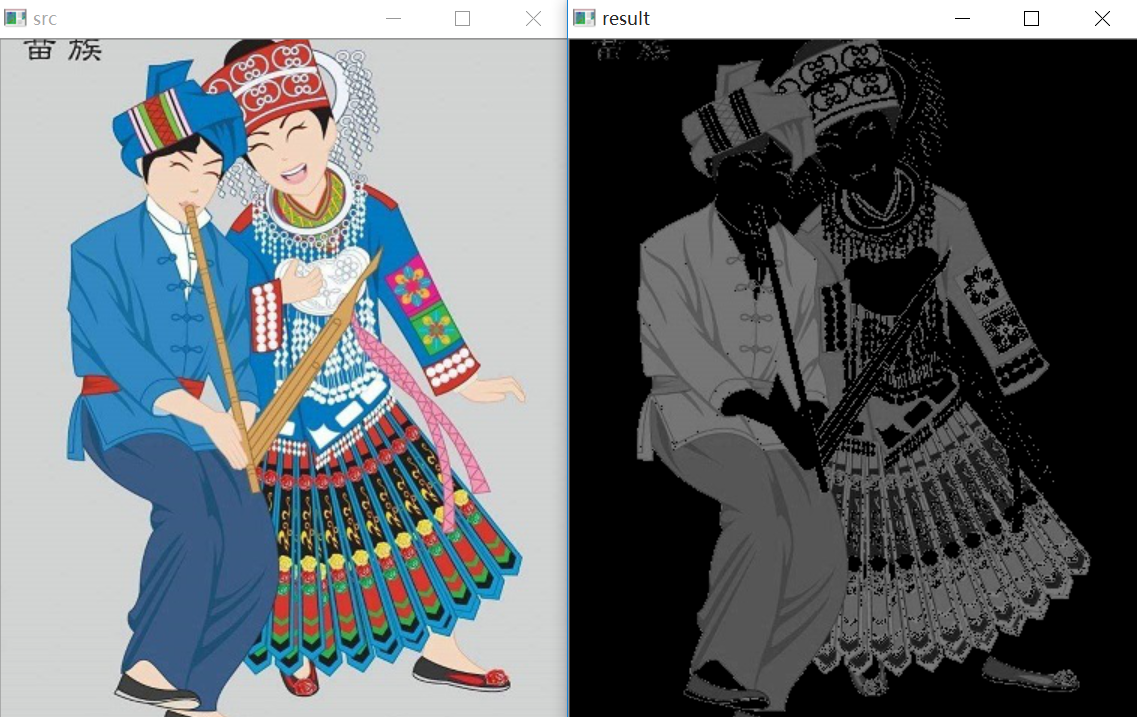六. 阈值化为0
该方法先选定一个阈值，比如127，接着对图像的灰度值进行如下处理：
$dst(x,y) = \begin{cases} src(x,y)， if src(x,y)>thresh\\ 0，otherwise\\ \end{cases}$
(1) 大于等于阈值127的像素点，值保持不变
(2) 小于该阈值的像素点值设置为0
例如，163->163，86->0，102->0，201->201。
关键字为 cv2.THRESH_TOZERO，完整代码如下：
#encoding:utf-8
import cv2
import numpy as np

#读取图片

#灰度图像处理
GrayImage = cv2.cvtColor(src,cv2.COLOR_BGR2GRAY)

#阈值化为0处理
r, b = cv2.threshold(GrayImage, 127, 255, cv2.THRESH_TOZERO)
print r

#显示图像
cv2.imshow("src", src)
cv2.imshow("result", b)

#等待显示
cv2.waitKey(0)
cv2.destroyAllWindows()

输出结果如下图所示：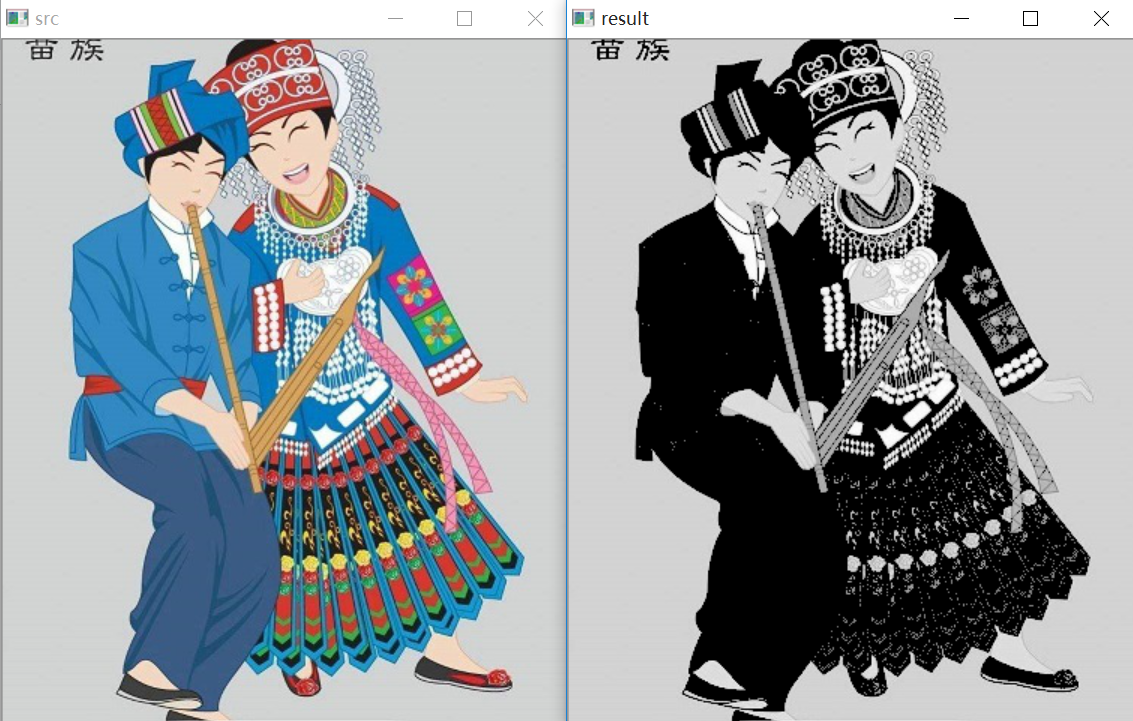该算法把比较亮的部分不变，比较暗的部分处理为0。
完整五个算法的对比代码如下所示：
#encoding:utf-8
import cv2
import numpy as np
import matplotlib.pyplot as plt

#读取图像
lenna_img = cv2.cvtColor(img,cv2.COLOR_BGR2RGB)
GrayImage=cv2.cvtColor(img,cv2.COLOR_BGR2GRAY)

#阈值化处理
ret,thresh1=cv2.threshold(GrayImage,127,255,cv2.THRESH_BINARY)
ret,thresh2=cv2.threshold(GrayImage,127,255,cv2.THRESH_BINARY_INV)
ret,thresh3=cv2.threshold(GrayImage,127,255,cv2.THRESH_TRUNC)
ret,thresh4=cv2.threshold(GrayImage,127,255,cv2.THRESH_TOZERO)
ret,thresh5=cv2.threshold(GrayImage,127,255,cv2.THRESH_TOZERO_INV)

#显示结果
titles = ['Gray Image','BINARY','BINARY_INV','TRUNC','TOZERO','TOZERO_INV']
images = [GrayImage, thresh1, thresh2, thresh3, thresh4, thresh5]
for i in xrange(6):
plt.subplot(2,3,i+1),plt.imshow(images[i],'gray')
plt.title(titles[i])
plt.xticks([]),plt.yticks([])
plt.show()

输出结果如下图所示：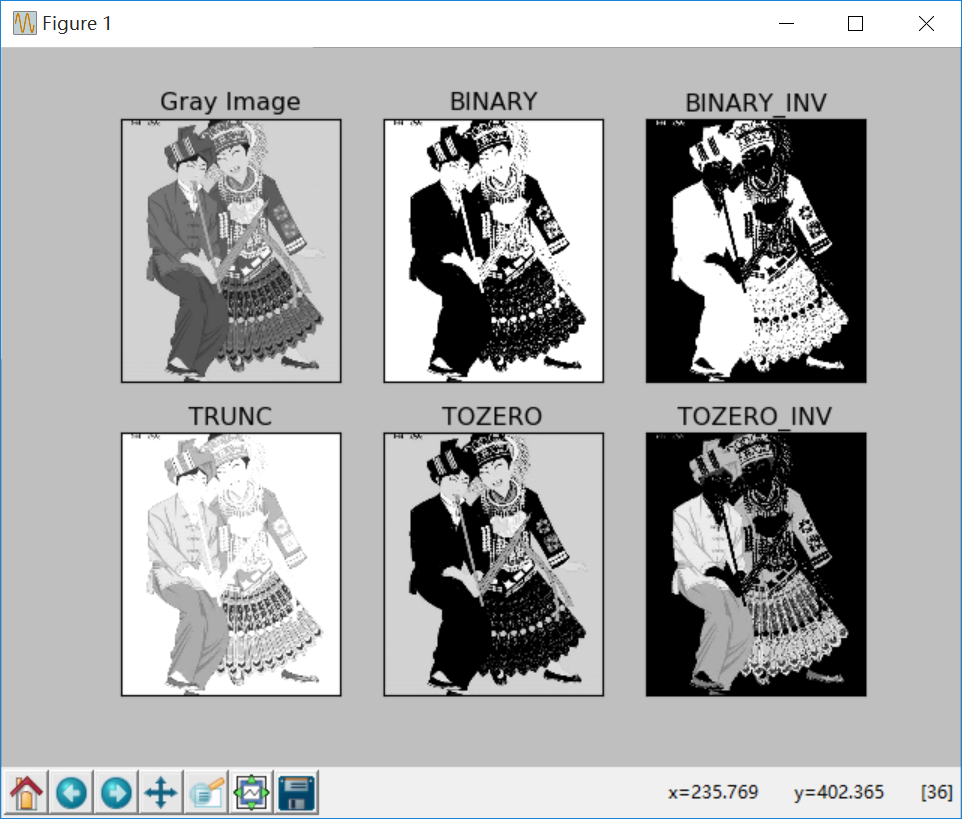希望文章对大家有所帮助，如果有错误或不足之处，还请海涵。最近经历的事情太多，有喜有悲，关闭了朋友圈，希望通过不断学习和写文章来忘记烦劳，将忧郁转换为动力。哎，总感觉自己在感动这个世界，帮助所有人，而自己却…谁有关心秀璋？晚安。
（By：Eastmount 2018-10-30 晚上10点 https://blog.csdn.net/Eastmount/）

2020年8月18新开的“娜璋AI安全之家”，主要围绕Python大数据分析、网络空间安全、人工智能、Web渗透及攻防技术进行讲解，同时分享CCF、SCI、南核北核论文的算法实现。娜璋之家会更加系统，并重构作者的所有文章，从零讲解Python和安全，写了近十年文章，真心想把自己所学所感所做分享出来，还请各位多多指教，真诚邀请您的关注！谢谢。展开全文Python 图像处理 OpenCV 阈值化处理 threshold
• 一、OTSU阈值化处理(非API实现) OTSU又称大津算法，是nobuyuki otsu于1979年提出的一种寻找图像阈值的最大类间方差算法。 OTSU算法的步骤如下:  (1)、统计灰度级[0,255]中每个像素在整幅图像中的个数。  (2)、计算...
一、OTSU阈值化处理(非API实现)
OTSU算法的步骤如下:
(1)、统计灰度级[0,255]中每个像素在整幅图像中的个数。
(2)、计算每个像素在整个灰度级的分布情况。
(3)、对整个灰度级遍历，计算当前灰度值下的前、背景类间概率。
(4)、计算出类内与类间方差下的对应的阈值。
1、非API方式实现OTSU算法:
代码如下:
#include <opencv2\opencv.hpp>
#include <opencv2\highgui\highgui.hpp>
#include <stdio.h>
#include <string.h>
using namespace std;
using namespace cv;

int OTSU(Mat src)
{
int nRows = src.rows;
int nCols = src.cols;
int threshold = 0;
//初始化统计参数
int nSumpix;
float nDis;
for (int i = 0; i < 256; i++)
{
nSumpix[i] = 0;
nDis[i] = 0;
}
//统计灰度级中每个像素在整幅图的个数
for (int i = 0; i < nRows; i++)
{
for (int j = 0; j < nCols; j++)
{
nSumpix[(int)src.at<uchar>(i, j)]++;
}
}

//计算每个灰度级占图像中的分布
for (int i = 0; i < 256; i++)
{
nDis[i] = (float)nSumpix[i] / (nRows*nCols);
}

//遍历灰度级[0,255]，计算最大类方差的阈值
float w0, w1, u0_temp, u1_temp, u0, u1, delta;
double delta_max = 0.0;
for (int i = 0; i < 256; i++)
{
//初始化参数
w0 = w1 = u0_temp = u1_temp = u0 = u1 = delta = 0;
for (int j = 0; j < 256; j++)
{
if (j <= i)
{
//当前i为分割阈值
w0 += nDis[j];
u0_temp += j * nDis[j];
}
//前景部分
else
{
//当前i为分割阈值
w1 += nDis[j];
u1_temp += j * nDis[j];
}
}
//分别计算各类平均值
u0 = u0_temp / w0;
u1 = u1_temp / w1;
delta = (float)(w0*w1*pow((u0 - u1), 2));
//依次寻找最大类间方差的阈值
if (delta>delta_max)
{
delta_max = delta;
threshold = i;
}
}
return threshold;
}

int main(int argc, char* argv[])
{

if (!src.data)
return -1;
else
imshow("src", src);

Mat srcGray;
cvtColor(src, srcGray, CV_BGR2GRAY);
//调用UTSO得到阈值
int otsuThreshold = OTSU(srcGray);

Mat dst(srcGray.size(), CV_8UC1);

for (int i = 0; i < srcGray.rows; i++)
{
for (int j = 0; j < srcGray.cols; j++)
{
if (srcGray.at<uchar>(i, j) > otsuThreshold)
dst.at<uchar>(i, j) = 255;
else
dst.at<uchar>(i, j) = 0;
}
}
imshow("OTSU", dst);
waitKey(0);
return 0;
}


效果图:2、Opencv的API方式实现:
当然经典的算法也有加入到Opencv库中，下面即是直接调用API的方式实现OTSU阈值化操作:
使用的函数是：double threshold (InputArray src,  OutputArray dst,  double thresh,  double maxval,  int type)
src      --> 输入的图像(灰度图)
dst      --> 输出的图像
thresh --> 二值化的阈值
maxval-->用于设定THRESH_BINARY和THRESH_BINARY_INV阈值类型的最大阈值
#include <opencv2\opencv.hpp>
#include <opencv2\highgui\highgui.hpp>
using namespace std;
using namespace cv;

int main()
{
cvtColor(src, src, CV_BGR2GRAY);
Mat dst;
threshold(src, dst, 167, 255, THRESH_OTSU);
imshow("dst", dst);
waitKey();
return 0;
}上面函数就是采用的THRESH_OTSU方式，即Opencv封装好的大津算法的二值化。
当然，也可以添加一个TrackBar来实时调整阈值(当前为固定的167)。

二、其他方式的阈值化处理

THRESH_BINARY，表示dsti=(srci>T)?M:0
THRESH_BINARY_INV，表示dsti=(srci>T)?0:M
THRESH_TRUNC，表示dsti=(srci>T)?M:srci
THRESH_TOZERO_INV，表示dsti=(srci>T)?0:srci
THRESH_TOZERO，表示dsti=(srci>T)?srci:0

下面的两个图能直观的显示对应的阈值化类型的效果: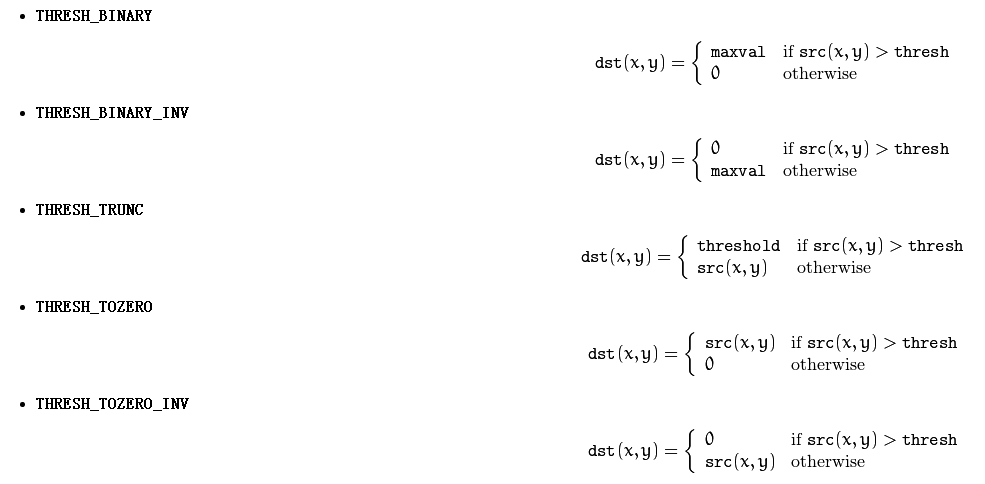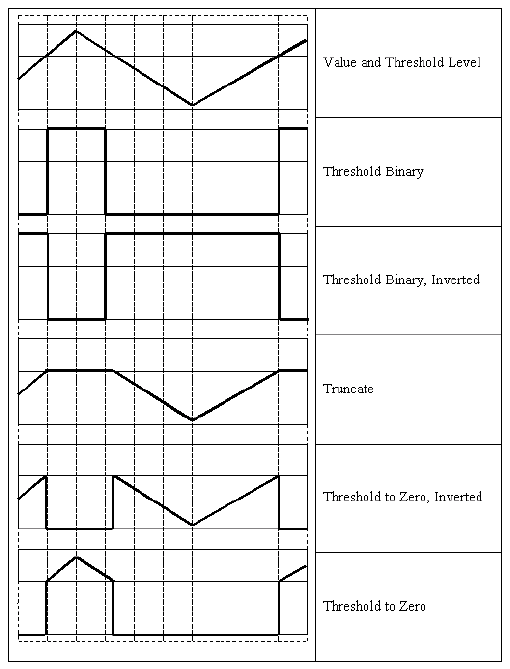代码以及效果图如下:

#include <opencv2\opencv.hpp>
#include <opencv2\highgui\highgui.hpp>
using namespace std;
using namespace cv;

int main()
{
imshow("src", src);
cvtColor(src, src, CV_BGR2GRAY);

Mat otsu;
threshold(src, otsu, 167, 255, THRESH_OTSU);
imshow("otsu", otsu);

Mat binary;
threshold(src, binary, 167, 255, THRESH_BINARY);
imshow("binary", binary);

Mat binary_inv;
threshold(src, binary_inv, 167, 255, THRESH_BINARY_INV);
imshow("binary_inv", binary_inv);

Mat trunc;
threshold(src, trunc, 167, 255, THRESH_TRUNC);
imshow("trunc", trunc);

Mat tozero_inv;
threshold(src, tozero_inv, 167, 255, THRESH_TOZERO_INV);
imshow("tozero_inv", tozero_inv);

Mat tozero;
threshold(src, tozero, 167, 255, THRESH_TOZERO);
imshow("tozero", tozero);

waitKey();
return 0;
}不同类型的阈值化有不同的效果: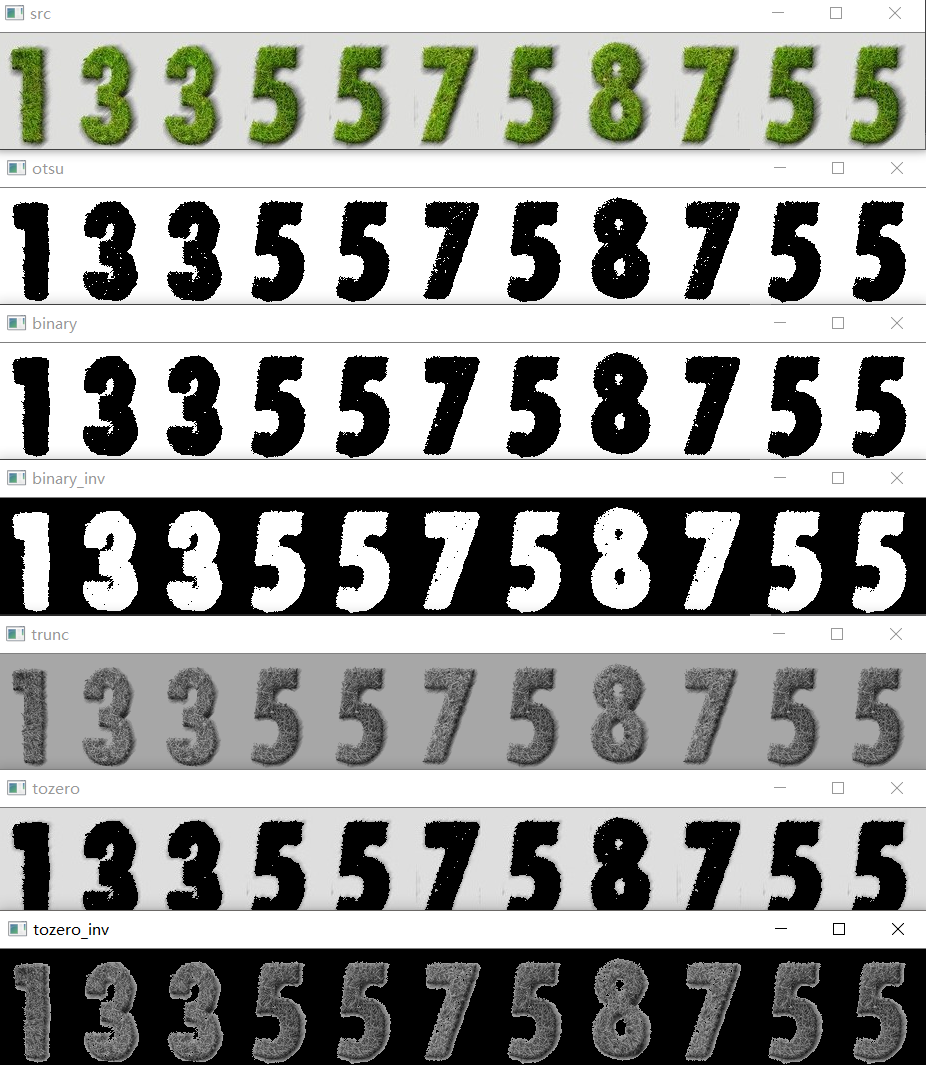在这里推荐一位大牛的主页:
Ce Liu:  Siftflow算法的作者，他个人主页上具有其他算法的源码供以学习。

鄙人才疏学浅，如有疏漏还望指正。


展开全文opencv
• 1 什么是阈值化-threshold() 2 二进制阈值化 3 反二进制阈值化 4 截断阈值化 5 反阈值化为0 6 阈值化为0 7 小结 参考资料 1 什么是阈值化-threshold() 图像的二值化或阈值化 (Binarization)旨在提取图像中...数字图像处理 Python
• 一、函数简介1、threshold—图像简单阈值化处理函数原型：threshold(src, thresh, maxval, type, dst=None)src：图像矩阵thresh：阈值maxVal：像素最大值type：阈值化类型2、adaptiveThreshold—图像自适应阈值化...
• 阈值可以被视作最简单的图像分割方法这样的图像分割方法基于图像中物体与背景之间的灰度差异，而且此分割属于像素级的分割。固定阈值操作–threshold()函数–单通道数组double threshold(inoutArray,outputArray,...opencv
• 在图像处理中，我们通常会...图像的阈值化处理是在灰度图像的基础上，把灰度图像进一步简化为二值图像，即整幅图像的各点像素值只用两个数值来表示，其中一个数值为零，另一个数值为非零值。图像的阈值化的关键是找...
• 功能很多，我们使用反二进制阈值化，作用有两点，一生成二值图像，二增强边缘图像的可视化效果。一、c++示例代码//包含头文件 #include &lt;opencv2/opencv.hpp&gt; //命名空间 using namespace cv; using ...opencv c++
• 所谓多维数组的阈值化处理，比如将矩阵中小于某阈值的元素全部置零。>> A = np.random.randn(3, 4) array([[-2.05480015, 1.02727696, -0.78219026, -1.77175268], [ 0.73452829, -0.27365904, -0.30674966, 0....
• Python-Opencv中阈值化操作和自适应阈值化，二值化操作 阈值化 阈值化图像其实就是对灰度图像进行二值化操作，根本原理是利用设定的阈值判断图像像素为0还是255，所以在图像二值化中阈值的设置很重要。图像的二值化...
• 一、函数简介1、threshold—图像简单阈值化处理函数原型：threshold(src, thresh, maxval, type, dst=None)src：图像矩阵thresh：阈值maxVal：像素最大值type：阈值化类型2、adaptiveThreshold—图像自适应阈值化...
• 阈值化是一种将我们想要在图像中分析的区域分割出来的方法。旨在提取图像中的目标物体，将背景以及噪声区分开来 二进制阈值化、反二进制阈值化、截断阈值化、反阈值化为0、阈值化为0 ...
• 图像阈值化 1、函数 （1）Python: cv2.cvtColor(src, code[, dst[, dstCn]]) → dst 箭头->dst表示函数运行输出一个结果dst. （2）Python: cv2.threshold(src, thresh, maxval, type[, dst]) → ...计算机视觉
• 图像分割  图像处理中很重要的概念就是图像分割，在很多应用都需要图像分割的处理，例如产品检测，目标识别，匹配等。图像分割的概念，我之前在...分别是边缘检测，阈值处理，基于区域的分割，还有其他的分割方法。
• 主要结合自己大三所学课程《数字图像处理》及课件进行讲解，主要通过MFC单文档视图实现显示BMP图片点运算处理，包括图像灰度线性变换、灰度非线性变换、图像阈值化处理、图像均衡化处理等知识，并结合前一篇论文灰度...数字图像处理 线性变换 图像均衡化
• 转自https://blog.csdn.net/eastmount/article/details/46312145本文主要讲述基于VC++6.0 ...包括图像灰度线性变换、灰度非线性变换、图像阈值化处理、图像均衡化处理等知识，并结合前一篇论文灰度直方图进行展示 。...
• 图像的阈值处理一般使得图像的像素值更单一、图像更简单。阈值可以分为全局性质的阈值，也可以分为局部性质的阈值，可以是单阈值的也可以是多阈值的。当然阈值越多是越复杂的。下面将介绍opencv下的三种阈值方法。...opencv python
...